Courses

# VSEPR Theory and Hybridisation JEE Notes | EduRev

## JEE : VSEPR Theory and Hybridisation JEE Notes | EduRev

The document VSEPR Theory and Hybridisation JEE Notes | EduRev is a part of the JEE Course Chemistry Class 11.
All you need of JEE at this link: JEE

VSEPR Theory

• The VSEPR theory is used to predict the shape of the molecules from the electron pairs that surround the central atoms of the molecule.
• The theory was first presented by Sidgwick and Powell in 1940. The two primary founders of the VSEPR theory are Ronald Nyholm and Ronald Gillespie. This theory is also known as the Gillespie-Nyholm theory to honour these chemists.
• The VSEPR theory is based on the assumption that the molecule will take a shape such that electronic repulsion in the valence shell of that atom is minimized.

What is VSEPR Theory?

• The Valence Shell Electron Pair Repulsion Theory, abbreviated as VSEPR theory, is based on the premise that there is a repulsion between the pairs of valence electrons in all atoms, and the atoms will always tend to arrange themselves in a manner in which this electron pair repulsion is minimalized. This arrangement of the atom determines the geometry of the resulting molecule.
• The different geometries that molecules can assume in accordance with the VSEPR theory can be seen in the illustration provided below: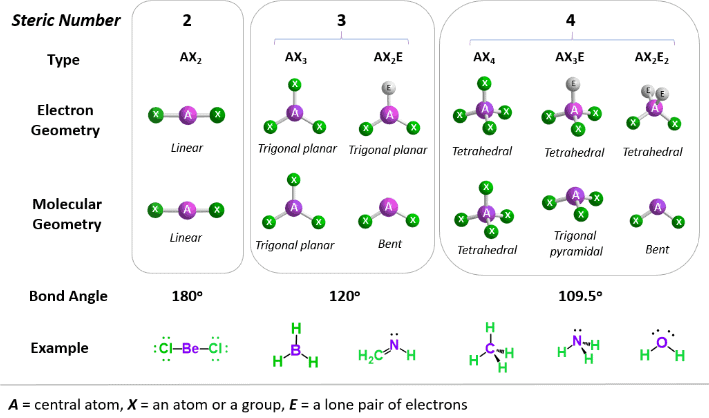• According to the VSEPR theory, the repulsion between two electrons is caused by the Pauli exclusion principle that has greater importance than electrostatic repulsion in the determination of molecular geometry.

Postulates of VSEPR Theory

The postulates of the VSEPR theory are listed below:

• In polyatomic molecules (i.e. molecules made up of three or more atoms), one of the constituent atoms is identified as the central atom to which all other atoms belonging to the molecule are linked.
• The total number of valence shell electron pairs decides the shape of the molecule.
• The electron pairs have a tendency to orient themselves in a way that minimizes the electron-electron repulsion between them and maximizes the distance between them.
• The valence shell can be thought of as a sphere wherein the electron pairs are localized on the surface in such a way that the distance between them is maximized.
• Should the central atom of the molecule be surrounded by bond pairs of electrons, then, the asymmetrically shaped molecule can be expected.
• Should the central atom be surrounded by both lone pairs and bond pairs of electrons, the molecule would tend to have a distorted shape.
• The VSEPR theory can be applied to each resonance structure of a molecule.
• The strength of the repulsion is strongest in two lone pairs and weakest in two bond pairs.
• If electron pairs around the central atom are closer to each other, they will repel each other. This results in an increase in the energy of the molecules.
• If the electron pairs lie far from each other, the repulsions between them will be less and eventually, the energy of the molecule will be low.

Limitations of VSEPR Theory

Some significant limitations of the VSEPR theory include:

• This theory fails to explain isoelectronic species (i.e. elements having the same number of electrons). The species may vary in shapes despite having the same number of electrons.
• The VSEPR theory does not shed any light on the compounds of transition metals. The structure of several such compounds cannot be correctly described by this theory. This is because the VSEPR theory does not take into account the associated sizes of the substituent groups and the lone pairs that are inactive.
• Another limitation of VSEPR theory is that it predicts that halides of group 2 elements will have a linear structure, whereas their actual structure is a bent one.

Predicting the Shapes of Molecules

The following steps must be followed in order to decide the shape of a molecule:

(i) The least electronegative atom must be selected as the central atom (since this atom has the highest ability to share its electrons with the other atoms belonging to the molecule).
(ii) The total number of electrons belonging to the outermost shell of the central atom must be counted.
(iii) The total number of electrons belonging to other atoms and used in bonds with the central atom must be counted.
(iv) These two values must be added in order to obtain the valence shell electron pair number or the VSEP number.

What is VSEP Number?

The VSEP number describes the shape of the molecule, as described in the table provided below: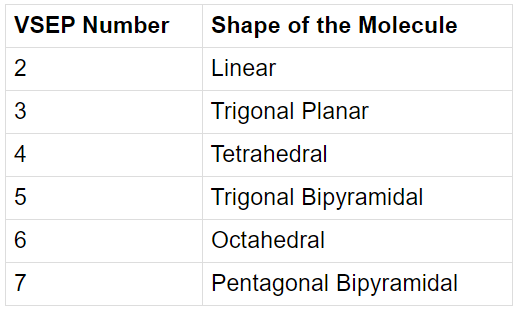The VSEPR theory cannot be used to obtain the exact bond angles between the atoms in a molecule.
Now, we will discuss each shape in detail:

1. Linear Shape of Molecule

• In this type of molecule, we find two places in the valence shell of the central atom.
• They should be arranged in such a manner such that repulsion can be minimized (pointing in the opposite direction).
• Example: BeF2Molecular structure of BeF2

2. Trigonal Planar Shape of Molecule

• In this type of molecule, we find three molecules attached to a central atom.
• They are arranged in such a manner such that repulsion between the electrons can be minimized (toward the corners of an equilateral triangle).
• Example: BF3Molecular structure of BF3

3. Tetrahedral Shape of Molecule

• In two-dimensional molecules, atoms lie in the same plane and if we place these conditions on methane, we will get a square planar geometry in which the bond angle between H-C-H is 90º.
• Now, if we consider all these conditions for a three-dimensional molecule, we will get a tetrahedral molecule in which the bond angle between H-C-H is 109º28’ (toward the corners of an equilateral triangle) CH4.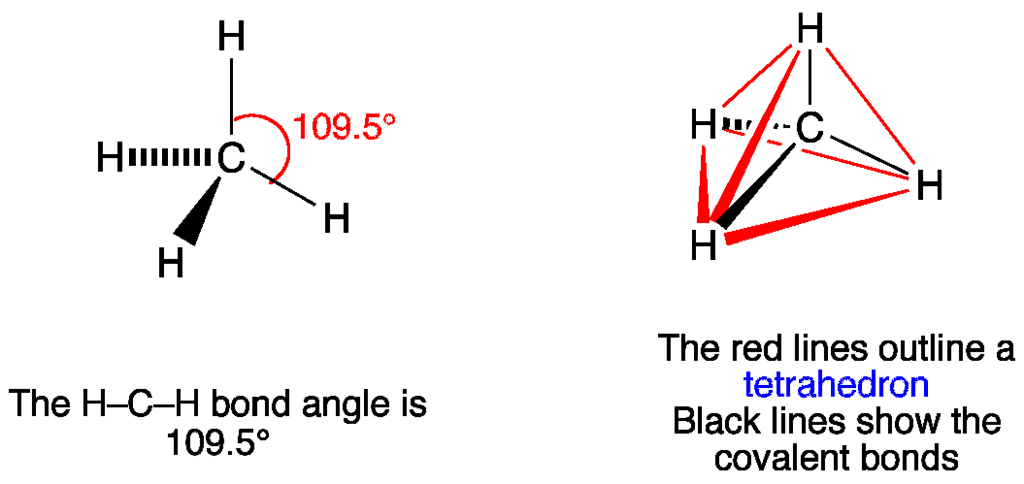Molecular Structure of CH4

4. Trigonal Bipyramid Shape of Molecule

• For PF5, repulsion can be minimized by even distribution of electrons towards the corner of a trigonal pyramid.
• In trigonal bipyramid, three positions lie along the equator of the molecule. The two positions lie along an axis perpendicular to the equatorial plane.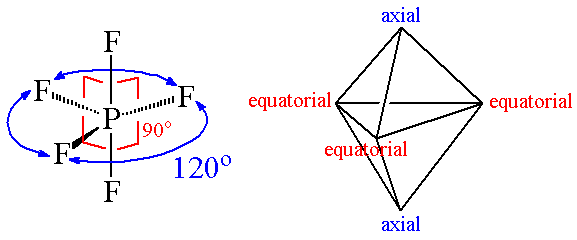Molecular structure of PF5

How can the VSEPR Theory be used to Predict the Shapes of Molecules?

• The strength of the repulsion between a lone pair and a bond pair of electrons lies in between the repulsion between two lone pairs and between two bond pairs.
• The order of repulsion between electron pairs is as follows:
Lone Pair- lone pair > Lone Pair- bond- pair > Bond Pair- bond pair.

(i) Total number of electron pairs around the central atom = ½ (number of valence electrons of central atom + number of atoms linked to central atom by single bonds)

• For negative ions, add the number of electrons equal to the units of negative charge on the ions to the valence electrons of the central atom.
• For positive ions, subtract the number of electrons equal to the units of positive charge on the ion from the valence electrons of the central atom.

(ii) The number of Bond pair = Total number of atoms linked to central atom by single bonds.
(iii) Number of lone pairs = Total number of electron – No of shared pair

The electron pairs around the central atom repel each another and move so far apart from each another that there are no greater repulsions between them. This results in the molecule having minimum energy and maximum stability.

• The shape of a molecule with only two atoms is always linear.
• For molecules with three or more atoms, one of the atoms is called the central atom and other atoms are attached to the central atom.
• If the central atom is linked to similar atoms and is surrounded by bond pairs of electrons only, the repulsions between them are similar as a result the shape of the molecule is symmetrical and the molecule is said to have regular geometry.
• If the central atom is linked to different atoms or is surrounded by bond pair as well as a lone pair of electrons, the repulsion between them is similar. As a result, the shape of the molecule has an irregular or distorted geometry.
• The exact shape of the molecule depends upon the total number of electron pairs present around the central atom.
Hybridisation

Hybridisation is defined as the mixing of the atomic orbitals belonging to the same atom but having slightly different energies so that redistribution of energy takes place between them resulting in the formation of new orbitals of equal energies and identical shapes. The new orbitals thus formed are known as hybrid orbitals.

• Only those orbitals which have approximately equal energies and belong to the same atom or ion can undergo hybridisation.
• The number of hybrid orbitals produced is equal to the number of atomic orbitals mixed.
• It is not necessary that all the half-filled orbitals must participate in hybridisation. Similarly, it is not necessary that only half-filled orbitals should participate in hybridisation. Even completely filled orbitals with slightly different energies can also participate.
• Hybridisation never takes place in isolated atoms but it occurs only at the time of bond formation.
• Type of hybridisation indicates the geometry of molecules. One can tell the shape of a molecule by knowing the kind of hybridisation involved.
• The bigger lobe of the hybrid orbital always has +ve sign while the smaller lobe on the opposite side has a -ve sign.
Type of Hybridisation

1. Diagonal or sp hybridisation

• When one s and one p orbital belonging to the same main shell of an atom mix together to form two new equivalent orbitals, this type of hybridisation is called sp hybridisation or diagonal hybridisation.
• The new orbitals formed are called sp hybrid orbitals.2. Trigonal or sp2 hybridisation

• When one s and two p orbitals of the same shell of an atom mix to form three new equivalent orbitals, this type of hybridisation is called sp2 hybridisation or trigonal hybridisation.
• The new orbitals formed are called sp2 hybrid orbitals.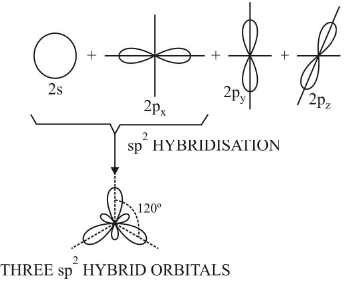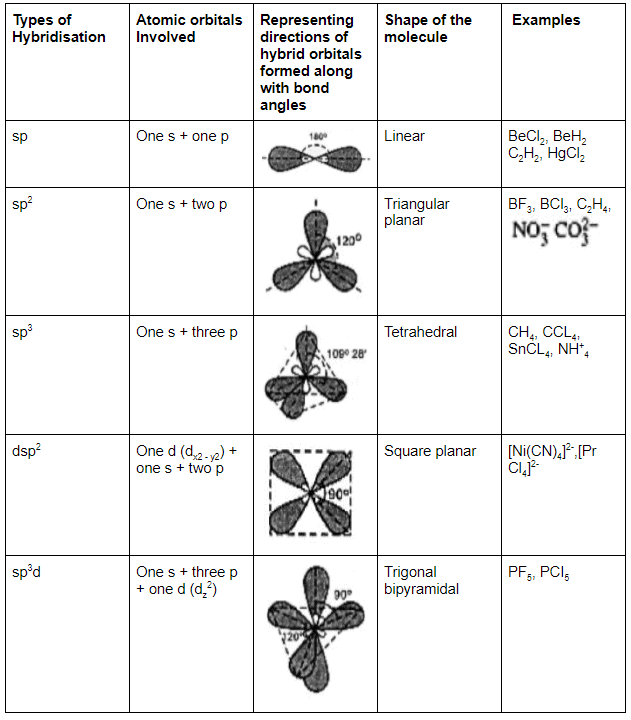Predicting Hybridisation

Calculate the number of hybrid orbitals (X) to be formed by the central atom as follows: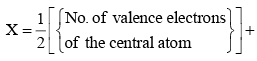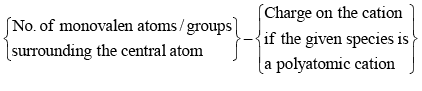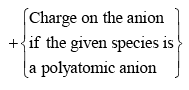i.e. X = 1/2 [ VE + MA - c + a]
Note that only monovalent atoms (MA) or groups are to be considered. For divalent ions, MA = 0.

• If X = 2, it means two hybrid orbitals are to be formed. Hence, hybridization is sp.
• If X = 3, it means three hybrid orbitals are to be formed. Hence, hybridisation is sp2
• And so on, as given in the following table: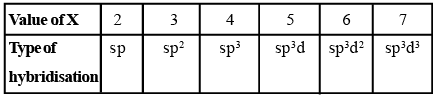Offer running on EduRev: Apply code STAYHOME200 to get INR 200 off on our premium plan EduRev Infinity!

## Chemistry Class 11

136 videos|245 docs|180 tests

,

,

,

,

,

,

,

,

,

,

,

,

,

,

,

,

,

,

,

,

,

;